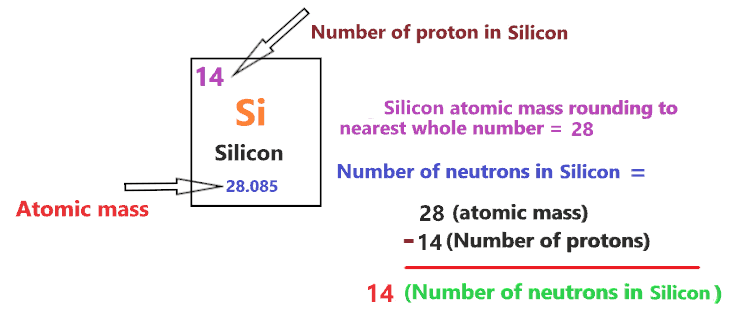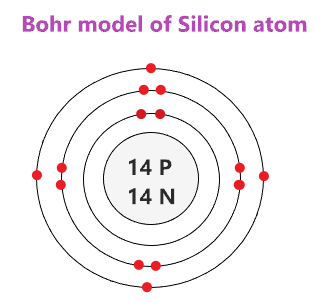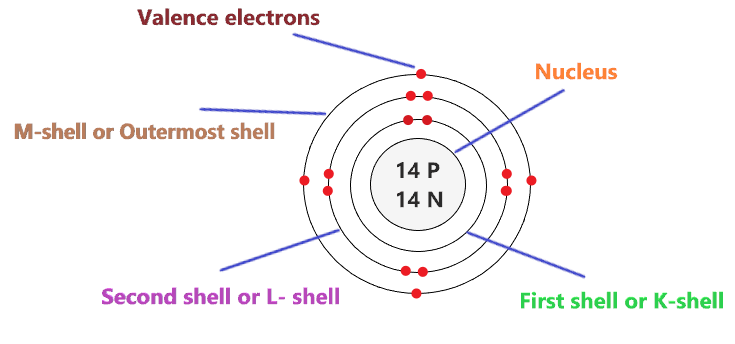# Bohr model of Silicon atom - How to draw Silicon(Si) Bohr-Rutherford diagram?

Home  > Chemistry Article > Silicon Bohr model

The Bohr Model of Silicon(Si) has a nucleus that contains 14 neutrons and 14 protons. This nucleus is surrounded by three-electron shells named K-shell, L-shell, and M-shell. The outermost shell in the Bohr diagram of Silicon contains 4 electrons that also called valence electrons.

 Name Silicon Bohr Model Number of neutrons 14 Number of protons 14 Number of electrons 14 Total electron shells 3 Electron in the First shell(K) 2 Electrons in the Second shell(L) 8 Electrons in the Third shell(M) 4 Total valence electrons in Silicon 4
Page Contents

## How to draw Bohr Model of Silicon(Si)?

Bohr model describes the visual representation of orbiting electrons around the small nucleus. It used different electron shells such as K, L, M, N…so on. These shells hold a specific number of electrons, the electron shell which is closest to the nucleus has less energy and the electron shell which is farthest from the nucleus has more energy.

Bohr diagram is very interesting and easy to draw. Here, we will draw the Bohr diagram of the Silicon atom with some simple steps.

## Steps to draw the Bohr Model of Silicon atom

1. Find the number of protons, electrons, and neutrons in the Silicon atom

Protons are the positively charged particles and neutrons are the uncharged particles, both these are constituents of the atom nuclei. Electrons are the negatively charged particles that orbit the nucleus of an atom

To find the number of protons an atom contains, just look at their atomic number.

If the atomic number of atom is 24, then proton will also be 24, if atomic number is 25, then proton will also be 25.

So, the atomic number for Silicon is 14, hence, the number of protons in the Silicon atom is also 14.Now, to determine the number of neutrons in an atom, use this formula.

⇒ Number of neutrons in atom = Atomic mass of the atom(rounded to the nearest whole number) – Number of proton in an atom

For example, An atom have 24.918 atomic mass and 12 protons.

Then, to find the number of neutron, round the atomic mass to the near whole number, so, atomic mass 24.918 round to 25.

= (25 – 12 protons) = 13 number of neutrons in the atom

Now, To get the number of neutrons in a Silicon atom, look at its atomic mass which is 28.085 rounded to 28, and the number of protons in Silicon is 14.

∴ Hence, the number of neutrons in Silicon atom = (28 – 14) = 14It should be noted that “The number of electrons in a neutral atom is equal to the number of protons”.

So, the Silicon atom is neutral, hence, its number of electrons will be equal to the number of protons which is 14 as we already discussed.

⇒ The number of electrons in Silicon atom = 14

⇒ The number of protons in Silicon atom = 14

⇒ The number of neutrons in Silicon atom = 14

2. Draw the nucleus of an atom

A nucleus is a dense and small region that contains the number of protons and neutrons of an atom.

In this step, we have to draw a small circle that consists of a number of protons and the number of neutrons of a Silicon atom.3. Draw the First electron shell

“An electron shell may be thought of as an orbit followed by electrons around an atom’s nucleus.”

The first electron shell is also called the K-shell, this is the closest shell to the nucleus of an atom and can hold a maximum of two electrons.

As we know, the Silicon atom has a total of 14 electrons. So, put two electrons from it, in the first shell, next to each other.We have successfully drawn the first shell of the Silicon atom that can hold 2 electrons. As a Silicon atom has a total of 14 electrons, and from 14 electrons we have used two electrons in the first shell.

∴ (14 – 2) = 12 electrons

Therefore, we are left with 12 electrons, let’s put them in the next shells of the Silicon atom.

4. Draw the Second electron shell

The second shell also called the L-shell that can hold a maximum of 8 electrons. This shell is drawn after the first electron shell.

In the second electron shell, the electrons are added one at a time, starting from the top position then going in a clockwise direction.

In second shell, electrons are added one at a time in clockwise direction as a clock position – 12 o’clock, 3 o’clock, 6 o’clock, 9 o’clock positions.Once you place the electrons one at a time to each of the four sides(Top – Right – Bottom – Left], start pairing or doubling them.

So, we have 12 remaining electrons of a Silicon atom, and the 2nd shell can only hold a maximum of 8 electrons.

Therefore, put the 8 electrons of the Silicon atom in the 2nd electron shell, start from the top position, put electrons one at a time, and go in a clockwise direction(Top – Right – Bottom – Left). And, finally, pair them up.As we have a total of 14 electrons for the Silicon atom and we placed 2 electrons in the first shell and 8 electrons in the second shell, hence, we are left with 4 electrons more.

Let’s place it in the next shell.

5. Draw the Third electron shell

The third shell is also called the M-shell and it has the capacity to hold up to 18 electrons but, for the elements in the first few periods, the third shell only holds up to 8 electrons.

“The third shell can be considered to hold 8 or 18 electrons but in total the third shell can hold 18 electrons.”

For the first few elements, say, till atomic number 20 on the periodic table, the third shell holds up to 8 electrons.

⇒ For atomic number 20 or less than 20, the third shell can hold up to maximum of 8 electrons.

⇒ For the atomic number more than 20, say, atomic numbers 21, 22, 23, 24, 25, 26, 27,…….etc., the third shell is filled with more than 8 electrons and up to maximum of 18 electrons.

For the Silicon atom, the third shell is filled with the same procedure as the second shell.

So, we have left with only 4 electrons of a Silicon atom, so, start from the top position of the third shell, put electrons one at a time, and go in a clockwise direction(Top – Right – Bottom – Left).### Bohr model of Silicon atom

That’s all, this is our Bohr model of the Silicon atom that contains 14 protons and 14 neutrons in the nucleus region, and 14 electrons are orbited around the nucleus, two electrons in the first shell, eight electrons in the second shell, and four electrons in the third shell.

## Find Valence electron of Silicon through its Bohr diagram

Valence electrons are found in the outermost shell of an atom and they can take participate in the formation of a chemical bond. These electrons have more energy compare to the inner shell electrons.

From the Bohr diagram of an atom, we can easily find the number of valence electrons in an atom by looking at its outermost shell.

So, we have to find a valence electron in the Silicon atom, for this, look at its Bohr diagram.

Bohr’s diagram of Silicon has three electron shells (K, L, and M), the inner shell is K-shell and the outermost shell is M-shell.

Hence, the electrons found in the M-shell of the Silicon atom are its valence electrons because it is the outermost shell that also called the valence shell.

The M-shell or outer shell of the Silicon Bohr model contains 4 electrons, therefore, the number of the valence electrons in the Silicon atom is also 4.## Electron dot diagram of a Silicon atom

Electron dot diagram also called lewis structure which represents the valence electrons of atoms.

As, from the Bohr diagram of Silicon, we got to know, it has only 4 valence electrons.

So, just represent the 4 valence electrons around the Silicon atom as a dot.## The electron configuration of Silicon

Electron configuration is the distribution of electrons of an atom or molecule in atomic or molecule orbitals”

Silicon has an atomic number of 14 and it contains a total number of 14 electrons. From the Bohr model of Silicon, we know, 2 electrons are in the K-shell, 8 electrons are in the L-shell, and 4 electrons are in the M-shell.

So, based on the shell, the electronic configuration of the Silicon atom is [2, 8, 4].

Or the electronic configuration of Silicon is 1s22s22p63s23p2 since it contains a total of 14 electrons.## FAQ

### How many electron shells a Silicon Bohr model contains?

Electron shell also called energy level, you can find the number of electron shells for an element by knowing its period number in the periodic table.

The elements or atoms in the first period of the periodic table have one energy level or one electron shell, same as, the elements in the second period have two energy levels or two-electron shells and so on…

So, the Silicon atom belongs to the 3rd Period in the periodic table, hence, the number of electron shells for the Bohr model of Silicon will be 3 (K-shell, L-shell, and M-shell).

### What is the outer shell of the Bohr diagram of the Silicon atom?

The outermost shell also called the valence shell, which contains valence electrons of the atom.

According to the Bohr diagram of Silicon, the outer shell is M-shell which contains only 4 valence electrons.

## Properties of Silicon

1. It appears as a brittle crystalline solid with a blue-grey metallic luster.
2. It has a face-centered diamond-cubic crystal structure.
3. It has a boiling point of 3265 °C and a melting point of 1414 °C.
4. It is the second most abundant element in the Earth’s crust.
5. It has an electronegativity of 1.90 at the Pauling scale.

## Summary

• The Bohr model of Silicon is drawn with three electron shells, the first shell contains 2 electrons, the second shell contains 8 electrons and the third shell contains 4 electrons.
• Silicon is neutral and its atomic number is 14, hence, the number of protons and electrons available for its Bohr diagram is also 14.
• The number of neutrons for the Bohr diagram of Silicon can be found by subtracting the number of protons from the atomic mass(rounded to the nearest whole).
• The electron configuration of Silicon in terms of the shell is [2, 8, 4], or in normal form, it is [Ne] 3s23p2.
##### Subscribe to Blog via Email

Join 2 other subscribers

Share it...